# Algorithms and Flowcharts

17. Apr 2013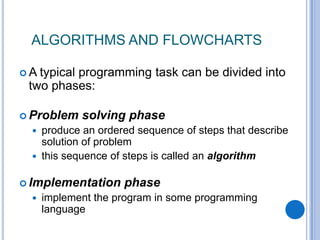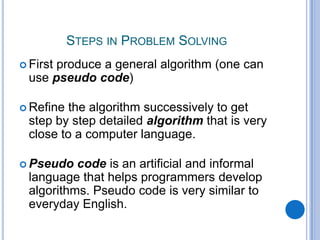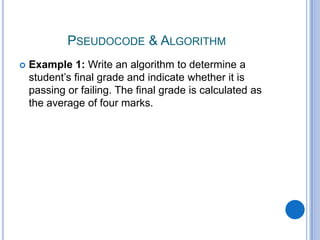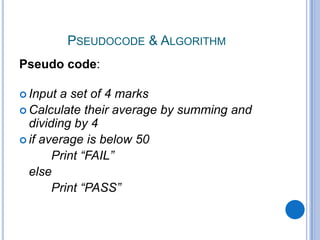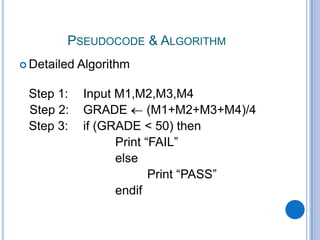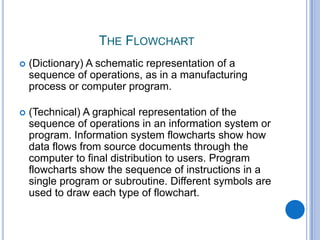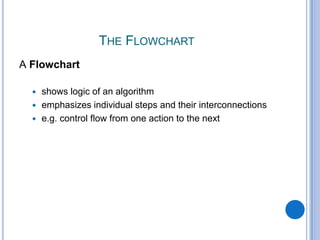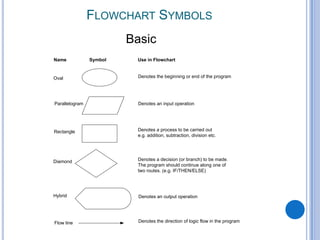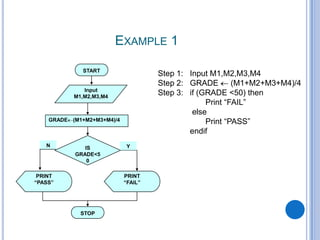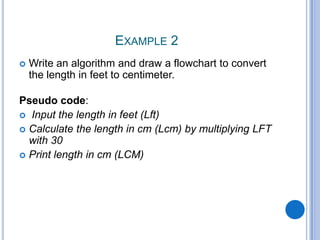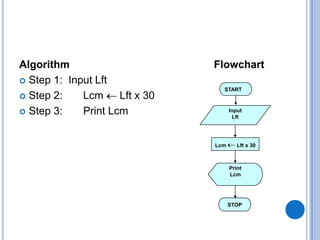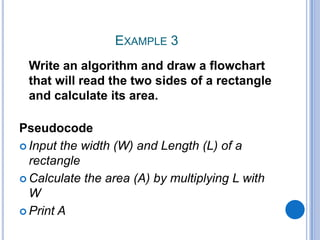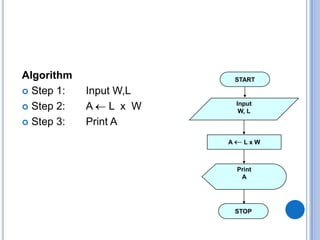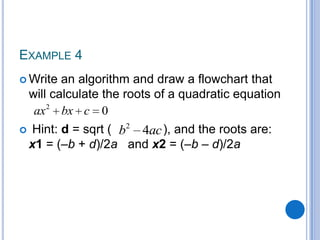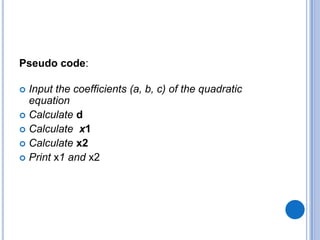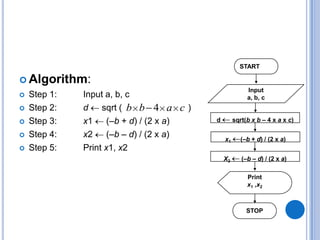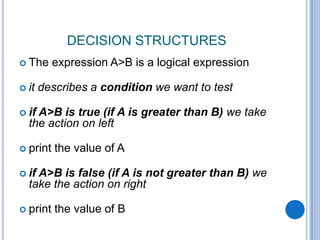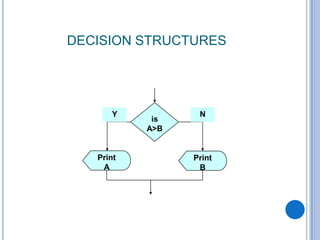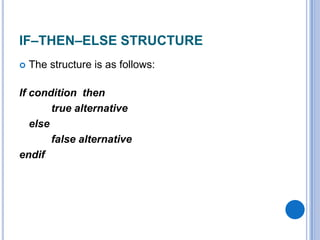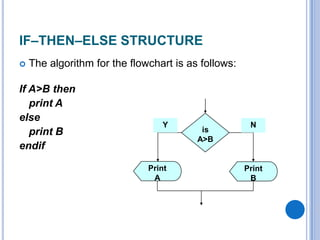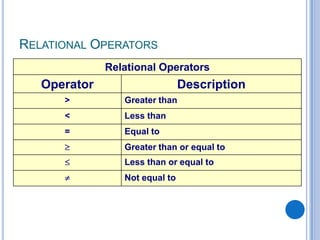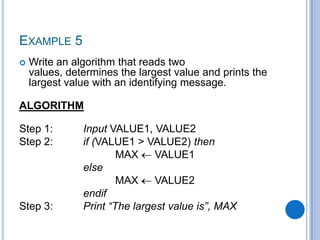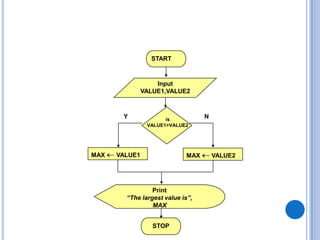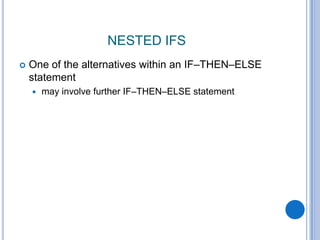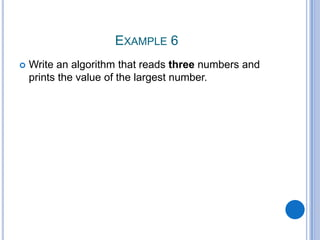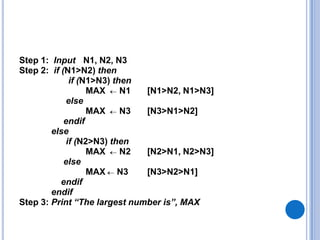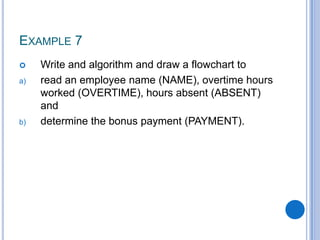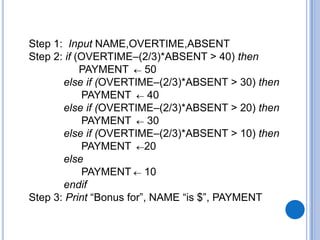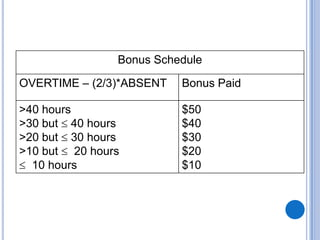1 von 31

### Algorithms and Flowcharts

• 1. COMPUTER SCIENCE COVERED BASICS ABOUT ALGORITHMS AND FLOWCHARTS
• 2. ALGORITHMS AND FLOWCHARTS  A typical programming task can be divided into two phases:  Problem solving phase  produce an ordered sequence of steps that describe solution of problem  this sequence of steps is called an algorithm  Implementation phase  implement the program in some programming language
• 3. STEPS IN PROBLEM SOLVING  First produce a general algorithm (one can use pseudo code)  Refine the algorithm successively to get step by step detailed algorithm that is very close to a computer language.  Pseudo code is an artificial and informal language that helps programmers develop algorithms. Pseudo code is very similar to everyday English.
• 4. PSEUDOCODE & ALGORITHM  Example 1: Write an algorithm to determine a student’s final grade and indicate whether it is passing or failing. The final grade is calculated as the average of four marks.
• 5. PSEUDOCODE & ALGORITHM Pseudo code:  Input a set of 4 marks  Calculate their average by summing and dividing by 4  if average is below 50 Print “FAIL” else Print “PASS”
• 6. PSEUDOCODE & ALGORITHM  Detailed Algorithm Step 1: Input M1,M2,M3,M4 Step 2: GRADE (M1+M2+M3+M4)/4 Step 3: if (GRADE < 50) then Print “FAIL” else Print “PASS” endif
• 7. THE FLOWCHART  (Dictionary) A schematic representation of a sequence of operations, as in a manufacturing process or computer program.  (Technical) A graphical representation of the sequence of operations in an information system or program. Information system flowcharts show how data flows from source documents through the computer to final distribution to users. Program flowcharts show the sequence of instructions in a single program or subroutine. Different symbols are used to draw each type of flowchart.
• 8. THE FLOWCHART A Flowchart  shows logic of an algorithm  emphasizes individual steps and their interconnections  e.g. control flow from one action to the next
• 9. FLOWCHART SYMBOLS Oval Parallelogram Rectangle Diamond Hybrid Name Symbol Use in Flowchart Denotes the beginning or end of the program Denotes an input operation Denotes an output operation Denotes a decision (or branch) to be made. The program should continue along one of two routes. (e.g. IF/THEN/ELSE) Denotes a process to be carried out e.g. addition, subtraction, division etc. Flow line Denotes the direction of logic flow in the program Basic
• 10. EXAMPLE 1 PRINT “PASS” Step 1: Input M1,M2,M3,M4 Step 2: GRADE (M1+M2+M3+M4)/4 Step 3: if (GRADE <50) then Print “FAIL” else Print “PASS” endif START Input M1,M2,M3,M4 GRADE (M1+M2+M3+M4)/4 IS GRADE<5 0 PRINT “FAIL” STOP YN
• 11. EXAMPLE 2  Write an algorithm and draw a flowchart to convert the length in feet to centimeter. Pseudo code:  Input the length in feet (Lft)  Calculate the length in cm (Lcm) by multiplying LFT with 30  Print length in cm (LCM)
• 12. Algorithm  Step 1: Input Lft  Step 2: Lcm Lft x 30  Step 3: Print Lcm START Input Lft Lcm Lft x 30 Print Lcm STOP Flowchart
• 13. EXAMPLE 3 Write an algorithm and draw a flowchart that will read the two sides of a rectangle and calculate its area. Pseudocode  Input the width (W) and Length (L) of a rectangle  Calculate the area (A) by multiplying L with W  Print A
• 14. Algorithm  Step 1: Input W,L  Step 2: A L x W  Step 3: Print A START Input W, L A L x W Print A STOP
• 15. EXAMPLE 4  Write an algorithm and draw a flowchart that will calculate the roots of a quadratic equation  Hint: d = sqrt ( ), and the roots are: x1 = (–b + d)/2a and x2 = (–b – d)/2a 2 0ax bx c 2 4b ac
• 16. Pseudo code:  Input the coefficients (a, b, c) of the quadratic equation  Calculate d  Calculate x1  Calculate x2  Print x1 and x2
• 17.  Algorithm:  Step 1: Input a, b, c  Step 2: d sqrt ( )  Step 3: x1 (–b + d) / (2 x a)  Step 4: x2 (–b – d) / (2 x a)  Step 5: Print x1, x2 4b b a c START Input a, b, c d sqrt(b x b – 4 x a x c) Print x1 ,x2 STOP x1 (–b + d) / (2 x a) X2 (–b – d) / (2 x a)
• 18. DECISION STRUCTURES  The expression A>B is a logical expression  it describes a condition we want to test  if A>B is true (if A is greater than B) we take the action on left  print the value of A  if A>B is false (if A is not greater than B) we take the action on right  print the value of B
• 20. IF–THEN–ELSE STRUCTURE  The structure is as follows: If condition then true alternative else false alternative endif
• 21. IF–THEN–ELSE STRUCTURE  The algorithm for the flowchart is as follows: If A>B then print A else print B endif is A>B Print B Print A Y N
• 22. RELATIONAL OPERATORS Relational Operators Operator Description > Greater than < Less than = Equal to Greater than or equal to Less than or equal to Not equal to
• 23. EXAMPLE 5  Write an algorithm that reads two values, determines the largest value and prints the largest value with an identifying message. ALGORITHM Step 1: Input VALUE1, VALUE2 Step 2: if (VALUE1 > VALUE2) then MAX VALUE1 else MAX VALUE2 endif Step 3: Print “The largest value is”, MAX
• 24. MAX VALUE1 Print “The largest value is”, MAX STOP Y N START Input VALUE1,VALUE2 MAX VALUE2 is VALUE1>VALUE2
• 25. NESTED IFS  One of the alternatives within an IF–THEN–ELSE statement  may involve further IF–THEN–ELSE statement
• 26. EXAMPLE 6  Write an algorithm that reads three numbers and prints the value of the largest number.
• 27. Step 1: Input N1, N2, N3 Step 2: if (N1>N2) then if (N1>N3) then MAX N1 [N1>N2, N1>N3] else MAX N3 [N3>N1>N2] endif else if (N2>N3) then MAX N2 [N2>N1, N2>N3] else MAX N3 [N3>N2>N1] endif endif Step 3: Print “The largest number is”, MAX
• 28. EXAMPLE 7  Write and algorithm and draw a flowchart to a) read an employee name (NAME), overtime hours worked (OVERTIME), hours absent (ABSENT) and b) determine the bonus payment (PAYMENT).
• 29. Step 1: Input NAME,OVERTIME,ABSENT Step 2: if (OVERTIME–(2/3)*ABSENT > 40) then PAYMENT 50 else if (OVERTIME–(2/3)*ABSENT > 30) then PAYMENT 40 else if (OVERTIME–(2/3)*ABSENT > 20) then PAYMENT 30 else if (OVERTIME–(2/3)*ABSENT > 10) then PAYMENT 20 else PAYMENT 10 endif Step 3: Print “Bonus for”, NAME “is \$”, PAYMENT
• 30. Bonus Schedule OVERTIME – (2/3)*ABSENT Bonus Paid >40 hours >30 but 40 hours >20 but 30 hours >10 but 20 hours 10 hours \$50 \$40 \$30 \$20 \$10